# The Use And Abuse Of The MDX Freeze Statement

The other day, while helping a customer with some particularly nasty MDX scoped assignments, I realised that there weren’t many good resources on the internet that explained how to use the MDX Freeze statement. It’s something I see used quite often, but usually because some MDX calculations aren’t giving the correct results and a developer has found that putting a Freeze statement in has fixed the problem – even if they don’t understand why it has fixed the problem. So, in this post I’ll explain what Freeze does, when you might want to use it, and when there are other other, better alternatives.

First of all, the basics. Imagine you have a super-simple cube and that, apart from the Calculate statement, the only MDX you have on the Calculations tab in the cube editor is the following:

```CREATE MEMBER CURRENTCUBE.MEASURES.M1 AS 1;

CREATE MEMBER CURRENTCUBE.MEASURES.M2 AS NULL;

SCOPE(MEASURES.M2);
THIS = MEASURES.M1;
END SCOPE;
```

If you query the cube in Excel, you’ll see the following: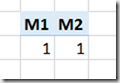No surprises here: we have created two calculated measures, M1 and M2, and then used a scoped assignment to set M2 to show the value of M1. It’s important to understand that the scope statement has not copied the value of M1 into M2, but acts more like a pointer so that M1 will always display the same value as M2 even if M1 subsequently changes. This means that when we add a second scope statement to the code that alters the value of M1, as follows:

```CREATE MEMBER CURRENTCUBE.MEASURES.M1 AS 1;

CREATE MEMBER CURRENTCUBE.MEASURES.M2 AS NULL;

SCOPE(MEASURES.M2);
THIS = MEASURES.M1;
END SCOPE;

SCOPE(MEASURES.M1);
THIS = 2;
END SCOPE;
```

You see the following in your PivotTable:This behaviour is the source of a lot of confusion! An assignment to one measure has indirectly changed the value of another measure, and of course in a real-world cube it can be very difficult to spot situations where this has happened and if you do, what other MDX has caused this to happen.

Each statement in the MDX Script of a cube adds an extra layer of calculations to it, called a calculation pass; this is true for all the calculations in the examples above. As new calculations are added, and new passes are created, the previous passes still exist and are still accessible. In the second example above, in the outermost calculation pass, the measure M2 returns the value 2 but at the previous calculation pass (as seen in the first example) it returned the value 1. The Freeze statement allows you to freeze the values returned by a subcube of cells at a given calculation pass, so that no future calculations will change those values.

Therefore, by taking our code and adding a Freeze statement to the first scoped assignment we can prevent the second scoped assignment changing the value of M2:

```CREATE MEMBER CURRENTCUBE.MEASURES.M1 AS 1;

CREATE MEMBER CURRENTCUBE.MEASURES.M2 AS NULL;

SCOPE(MEASURES.M2);
THIS = MEASURES.M1;
FREEZE(THIS);
END SCOPE;

SCOPE(MEASURES.M1);
THIS = 2;
END SCOPE;
```

Here’s the output now: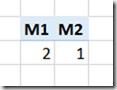Another very common way that scoped assignments can affect the value of a cell is through the aggregation of the results of a calculation. This blog post (one of the most popular I’ve ever written) explains how this behaviour can be used to implement calculations like currency conversions and weighted averages. However, in other cases, this aggregation of a calculation is an unwanted and unexpected side effect of a scope statement and calculated values that you did want to be displayed instead get replaced with weird, meaningless values. The Freeze statement can be used to stop this happening but in actual fact it’s a much better idea to understand the cause of these problems and rewrite your calculations so that Freeze isn’t necessary.

Now, imagine that in your cube you have a regular (ie not calculated) measure called Sales Amount that has its AggregateFunction property set to Sum, and that you have a fairly standard Date dimension with a Year attribute hierarchy. A PivotTable with Sales Amount on columns and Year on rows looks like this in Excel:If you add the following assignment to the cube, to change the value of the All Member on Year, the value of the Grand Total in the PivotTable (which is the All Member, even if that name isn’t shown) will be changed:

```SCOPE([Date].[Year].[All], [Measures].[Sales Amount]);
THIS = 123;
END SCOPE;
```If, on the other hand, you remove that previous assignment and replace it with an assignment on the year 2001:

```SCOPE([Date].[Year].&amp;, [Measures].[Sales Amount]);
THIS = 456;
END SCOPE;
```

You’ll see that not only has the value for Sales Amount for the year 2001 changed, but that the value of the All Member has been changed too: the All Member represents the aggregated total of all the years, so therefore if a year value has changed, the All Member value must change the reflect this: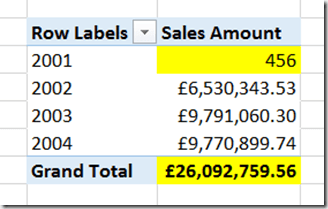What happens if we try to combine the two previous scope statements?

```SCOPE([Date].[Year].[All], [Measures].[Sales Amount]);
THIS = 123;
END SCOPE;

SCOPE([Date].[Year].&, [Measures].[Sales Amount]);
THIS = 456;
END SCOPE;
```

In this case, the output is exactly the same as with the previous example (although the measure formatting has also been lost):This is because even though the first Scope statement successfully changed the value of the All Member, the aggregation of values triggered by the second Scope overwrote this value. Although you can’t see this happening in Excel, where you only see the values returned at the final calculation pass of the cube, the MDX Script Debugger can be used to see the values returned for a query at all the different passes so you can work out what’s going on.

The Freeze statement can be used to stop the second Scope from overwriting the first, like so:

```SCOPE([Date].[Year].[All], [Measures].[Sales Amount]);
THIS = 123;
FREEZE(THIS);
END SCOPE;

SCOPE([Date].[Year].&, [Measures].[Sales Amount]);
THIS = 456;
END SCOPE;
```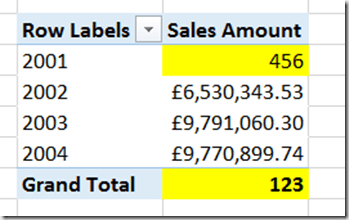However, in my opinion it makes a lot more sense to change the order of the Scope statements so that the assignment to 2001 doesn’t overwrite the assignment to the All Member:

```SCOPE([Date].[Year].&, [Measures].[Sales Amount]);
THIS = 456;
END SCOPE;

SCOPE([Date].[Year].[All], [Measures].[Sales Amount]);
THIS = 123;
END SCOPE;
```

The end result is the same: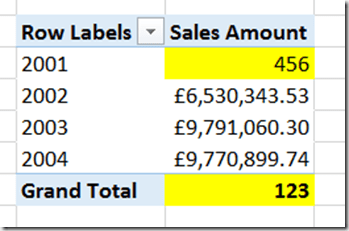Why do I prefer this approach to the use of Freeze? Two reasons:

1. It works with natural MDX behaviour rather than trying to fight against it. In this case it’s just one line of code less, but in the real world it could result in a much greater reduction. It’s true that you have to put a lot of thought into the ordering of your calculations, but I don’t think you can get away from that. Using Freeze to make your calculations work properly without understanding why it’s needed results in much more complex code, often with duplicated calculations because Freeze still doesn’t give the desired results, and is frankly a bit of a hack.
2. There are, or at least were, performance implications with the use of Freeze. In Analysis Services 2005 I saw a few cases where the use of Freeze contributed to poor query performance, and where reordering scope statements so that it was no longer necessary made performance better. I’m not sure whether this is still the case with SSAS 2014 but it may well be.

I see Freeze abused most often in financial cubes, when scope statements are used to define calculations on a chart of accounts hierarchy. Sometimes I have even seen the same calculation code appear in several places in the same MDX Script, just to make sure that the calculations always return the right result – all because the calculations on the chart of accounts dimension are aggregating up and overwriting each other. In this case the simple rule you have to remember is to always scope the calculations on the lowest level of the hierarchy first, then scope the calculations on the second-lowest level, and so on working your way up to the top of the hierarchy. This way you can be sure that your scope will never aggregate up and overwrite the result of another calculation.

Apart from that, I also see Freeze used when a cube contains a Date Tool dimension that uses regular members instead of calculated members, in the way described here. Now there are a lot of good reasons to use regular members on a Date Tool dimension (it will work with all versions of SSAS and Excel for instance) but I have also seen a lot of cases where the fact that you are scoping calculations on regular measures, which may then get aggregated up accidentally, has caused a lot of problems – not only resulting in incorrect values appearing, but also making query performance worse. For that reason, nowadays I prefer to use calculated members on my Date Tool dimension rather than regular members.

### 3 responses

1.Martin says:

Hi Chris,

Great article!

I have a question.

In one solution that i’ll work with, we have several freeze statements before some scope assignments in order to prevent the scoped members from aggregating up in the total.

We are scoping on regular members that are created in the dimension view.

Example below:

FREEZE [Receiving Country].[Receiving Country].[All];

scope ([Receiving Country].[Receiving Country].[Region].[Europe+Africa]);
this = [Receiving Country].[Receiving Country].[Region].[Europe]
+[Receiving Country].[Receiving Country].[Region].[ Africa];
end scope;

Will it work to re-write the logic with create member instead? If my understanding is correct this will prevent the member to aggregate up in the total?

Best Regards
Martin

•Chris Webb says:

Yes, you’re right – rewriting this as a calculated member will mean you can avoid using FREEZE.

•Martin says:

Thanks Chris, I will try to rewrite the Freeze statements.

//Martin

This site uses Akismet to reduce spam. Learn how your comment data is processed.